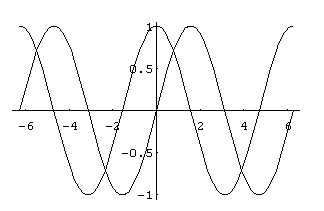```
```

# The "Plot" command in MATHEMATICA

```
```

The basic command for sketching the graph of a real-valued function of one variable in MATHEMATICA is

Plot[ f, {x,xmin,xmax} ]

which will draw the graph of y=f(x) over the closed interval [xmin,xmax] on the x-axis. More generally

Plot[ {f1, f2,...}, {x,xmin,xmax} ]

will represent in one picture the graphs of y=f1(x), y=f2(x), ... over the closed interval [xmin,xmax] on the x-axis. For example the output of

Plot[ {Sin[x], Cos[x]}, {x,-2*Pi,2*Pi} ]

is:To get a fancier output we might add some modifiers such as

Plot[ {Sin[x], Cos[x]}, {x,-2*Pi,2*Pi}, PlotStyle -> {Hue[.3], Hue[.8]},
PlotRange -> {-1.5, 1.5}, Frame -> True, AxesLabel -> {"x-axis","y-axis"} ]

obtaining:Here is a table describing some of the most useful modifiers for the PLOT command.

 AspectRatio -> NN control aspect ratio (proportions) of viewing window PlotRange -> {NN,NN} set the height of the window Axes -> BB include axes or not AxesLabel -> {"xlabel","ylabel"} label the axes PlotLabel -> "text for title" put title on graph Background -> Hue[NN] color the background PlotStyle -> {{s1},{s2},...} set the color and the "style" of curves GridLines -> Automatic add grid lines to graph

In this table, NN denotes a numerical value (which should be between 0 and 1 for Hue[NN]). The symbol BB can be one of the values True or False. And s1 may include specifications such as Hue[NN] (setting curve color), AbsoluteThickness[NN] (setting curve thickness), or Dashing[{NN,NN}] (making the curve dashed). The modifier AspectRatio->Automatic gives the visually true proportions (where the x- and y-axes are scaled equally).

Instead of Hue[NN], colors can also be specified with RGBColor[NN,NN,NN]. Desired colors may be previewed and chosen using the "Color Selector" from the "Input" menu.

To illustrate the "PlotStyle" modifier, the input

Plot[ {Sin[x],Cos[x]}, {x,-2*Pi,2*Pi},
PlotStyle -> { {Hue[.3],AbsoluteThickness}, {Hue[.8],Dashing[{.01,.01}]} } ]

will result in:If we want to add a frame to this picture, on the next input we could enter Show[ %, Frame->True ]. The effect of the "Show" command is to redraw the graphic (in this case the graphic in the previous output %) with the additional modifier Frame->True.```
```

URL: http://math.ou.edu/~amiller/math/plot.htm

August, 1999